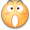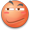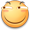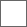• 19回复贴，共1

### 【转贴】仙剑奇侠传3模型文件格式分析

1、文件应该是没有压缩的，理由是文件中重复的字节很多，而且明显能看出很多的字符串。
2、文件的开头有个POLYd字样，应该是文件的合法性标识。打开其他的文件比较了一下，可以证实这个判断。
3、在文件开头有个Box02，可能是模型的名字。
4、紧跟模型名字有很多扩展名，作用不明，结束的位置也不清楚。
5、在这些扩展名之后紧跟了很多数据，含义未知。在这段区域中，每隔固定的长度都会出现 FFFF FFFF 字节。
6、在这段数据之后出现了一些重复的字节，之后是一个图像的文件名xiangzi.tga，应该是该模型的贴图。但是在众多资源中并没有找到这个贴图，现存疑。 这个字符串长度为11，但是在它前面没有指定这个长度的字节存在。其后又是一片空白的区域。
7、 最后存在一些数据区域。

1、文件开头有一个固定的标识。
2、之后是模型的名称和不明的数据
3、紧接着是顶点数据，每个顶点由 FFFF FFFF字节结束
4、然后是贴图文件名
5、最后是面数据，short型

while(最后面是 FFFF FFFF)
{

}

struct vertex
{
float u;
float v;
float x;
float y;
float z;
};

int faceCount;
struct face
{
short v1;
short v2;
short v3;
};

x+y=a;
x+3y=b;

{
float x;
float y;
float z;
int FFFFFFFF;
float u;
float v;
}

-文件标识
-不知道的内容
-文件所包含的模型数目
-不知道的内容

-顶点的数据
--顶点数据区的头
--每个顶点的数据
---顶点位置坐标
---FFFF FFFF
---顶点贴图坐标
-贴图数据（固定长度）
-面数据
--面数据头（包含了面的数目）
--面的数据

xj3Model.c#define _CRT_SECURE_NO_DEPRECATE

#include <iostream>
#include <vector>
using namespace std;

//定义文件头
{
//文件合法性判断
char valid;
//未知
int iDontKnow;
//模型计数
int polyCount;
};

//模型头
{
char iDontKnow;
};

//顶点头
{
char iDontKnow;
int vertexCount;
};

//顶点
struct vertex
{
//顶点的坐标
float x;
float y;
float z;
//FFFF FFFF
long e;
//顶点的贴图坐标
float u;
float v;
};

//贴图数据区
struct betweenVertexAndFace
{
//贴图数据区，固定长度
char iDontKnow;
};

//面数据区头
{
int iDontKnow;
int whoCares;
int faceCount;
};

//三角面数据
struct face
{
short a;
short b;
short c;
};

//入口
int main(int argc, char* argv[])
{
//建立文件头
//显示指定的文件名
printf("input:%s\n", argv);

//打开指定的文件
FILE *fp;
fp = fopen(argv, "rb");

//读取文件头
//如果文件合法
{
printf("这应该是一个正确的仙三POL文件\n");
}
else
{
//不合法退出
printf("这不是仙三POL文件\n");
getchar();
exit(0);
}

//读取模型头
for (int i = 0; i<theFileHeader.polyCount; i++)
{
}

//存储顶点和面数据的空间
vector<struct vertex> vertexList;
vector<struct face> faceList;

//遍历全部的模型
for (int i = 0; i<theFileHeader.polyCount; i++)
{
//清空存储空间
vertexList.clear();
faceList.clear();
//得到顶点头
printf("vertex count [%d]\n", tempVertexHeader.vertexCount);
//遍历全部的顶点
for (int e = 0; e<tempVertexHeader.vertexCount; e++)
{
//保存顶点的数据
struct vertex tempVertex;
fread(&tempVertex, sizeof(struct vertex), 1, fp);
vertexList.push_back(tempVertex);
}
//读取贴图数据
struct betweenVertexAndFace tempBetween;
fread(&tempBetween, sizeof(struct betweenVertexAndFace), 1, fp);
//得到面数据的头部
printf("face count [%d]\n", tempFaceHeader.faceCount);
//遍历所有的面
for (int e = 0; e<tempFaceHeader.faceCount; e++)
{
//保存数据
struct face tempFace;
fread(&tempFace, sizeof(struct face), 1, fp);
faceList.push_back(tempFace);
}
//生成obj格式的文件
char outName = { 0 };
sprintf(outName, "%s%dOut.obj", argv, i);
FILE *outFp;
outFp = fopen(outName, "w");
printf("%s\n", outName);
for (int e = 0; e<vertexList.size(); e++)
{
fprintf(outFp, "v %f %f %f \n", vertexList[e].x, vertexList[e].y, vertexList[e].z);
}

fprintf(outFp, "\n\n");

for (int e = 0; e<vertexList.size(); e++)
{
fprintf(outFp, "vt %f %f\n", vertexList[e].u, vertexList[e].v);
}

fprintf(outFp, "\n\ng model%d\n", i);

for (int e = 0; e<faceList.size(); e++)
{
fprintf(outFp, "f %d/%d %d/%d %d/%d\n", faceList[e].a + 1, faceList[e].a + 1, faceList[e].b + 1, faceList[e].b + 1, faceList[e].c + 1, faceList[e].c + 1);
}
fprintf(outFp, "\n\ng\n\n");
fclose(outFp);
}
fclose(fp);
printf("导出成功!\n");
getchar();
}不知不觉已经过去快三年了前来考古